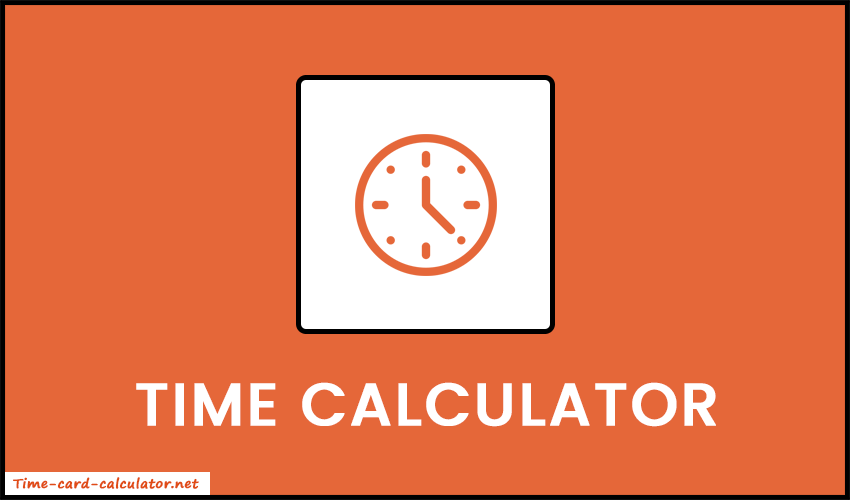# Time Calculator

Use our free time calculator to add or subtract two time values. Just input the values and press the Calculate button.

Time Card Calculator Time Duration Calculator

## What is Time Calculator?

The time calculator is an online web application that helps to calculate time in different formats. Using this tool, you can perform addition and subtraction between two times. Most commonly, it is used to calculate working hours and find the duration of any events or tasks. Also, it is very useful in accounting and project management.### How does the Time Calculator Work?

• It takes two time values from the users. The first one defines the start time and the second one defines the end time. Also, the input values should be in years, months, days, hours, minutes, and seconds.
• No need to enter all the values at a time. You can enter at least one value for the start time and one for the end time.
• In addition, our tool gives you the ability to choose the mathematical operation that you need to perform. Whether it's addition or subtraction, you can choose any one.
• Lastly, press the Calculate button to get the final result.